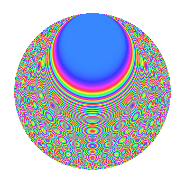# Properties

 Label 2100.2.tLevel 2100 Weight 2 Character orbit t Rep. character $$\chi_{2100}(43,\cdot)$$ Character field $$\Q(\zeta_{4})$$ Dimension 216 Sturm bound 960

# Related objects

## Defining parameters

 Level: $$N$$ $$=$$ $$2100 = 2^{2} \cdot 3 \cdot 5^{2} \cdot 7$$ Weight: $$k$$ $$=$$ $$2$$ Character orbit: $$[\chi]$$ $$=$$ 2100.t (of order $$4$$ and degree $$2$$) Character conductor: $$\operatorname{cond}(\chi)$$ $$=$$ $$20$$ Character field: $$\Q(i)$$ Sturm bound: $$960$$

## Dimensions

The following table gives the dimensions of various subspaces of $$M_{2}(2100, [\chi])$$.

Total New Old
Modular forms 1008 216 792
Cusp forms 912 216 696
Eisenstein series 96 0 96

## Trace form

 $$216q - 16q^{6} + O(q^{10})$$ $$216q - 16q^{6} - 16q^{12} - 8q^{13} - 16q^{16} - 40q^{17} + 24q^{22} + 40q^{32} + 16q^{36} + 8q^{37} + 56q^{38} + 56q^{46} + 16q^{52} + 8q^{53} + 24q^{56} - 32q^{58} - 128q^{61} - 40q^{62} + 56q^{68} + 104q^{73} + 128q^{76} - 24q^{78} - 216q^{81} + 96q^{82} + 104q^{86} + 8q^{88} + 40q^{92} - 16q^{93} - 64q^{96} + 40q^{97} + O(q^{100})$$

## Decomposition of $$S_{2}^{\mathrm{new}}(2100, [\chi])$$ into newform subspaces

The newforms in this space have not yet been added to the LMFDB.

## Decomposition of $$S_{2}^{\mathrm{old}}(2100, [\chi])$$ into lower level spaces

$$S_{2}^{\mathrm{old}}(2100, [\chi]) \cong$$ $$S_{2}^{\mathrm{new}}(20, [\chi])$$$$^{\oplus 8}$$$$\oplus$$$$S_{2}^{\mathrm{new}}(60, [\chi])$$$$^{\oplus 4}$$$$\oplus$$$$S_{2}^{\mathrm{new}}(100, [\chi])$$$$^{\oplus 4}$$$$\oplus$$$$S_{2}^{\mathrm{new}}(140, [\chi])$$$$^{\oplus 4}$$$$\oplus$$$$S_{2}^{\mathrm{new}}(300, [\chi])$$$$^{\oplus 2}$$$$\oplus$$$$S_{2}^{\mathrm{new}}(420, [\chi])$$$$^{\oplus 2}$$$$\oplus$$$$S_{2}^{\mathrm{new}}(700, [\chi])$$$$^{\oplus 2}$$

## Hecke characteristic polynomials

There are no characteristic polynomials of Hecke operators in the database